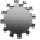Plenary Lecture

Generalized Symmetric Least-Squares RegressionsProfessor Nataniel Greene
Department of Mathematics and Computer Science
Kingsborough Community College
City University of New York
USA
E-mail: ngreene.math@gmail.com

Abstract: Ordinary least-squares regression suffers from a fundamental lack of symmetry: the inverse of the regression line of y given x is not the regression line of x given y. Alternative symmetric regression methods have been developed to address this concern, notably: orthogonal regression and geometric mean regression. In this talk a variety of symmetric least-squares regression methods are derived and analyzed, some of which may not have been known or fully explicated. A systematic approach to the derivation, analysis and classification of all symmetric least-squares methods is then described and a numerical procedure for comparing the various methods is presented.

Brief Biography of the Speaker: TBA